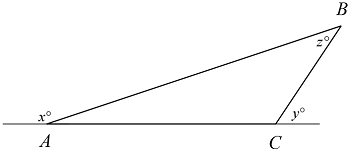# SAT Properties of TrianglesIn the figure above, which of the following is the value of $z$ expressed in terms of $x$ and $y?$

(A) $\ \ 180-x-y$
(B) $\ \ x+y+180$
(C) $\ \ x+y-180$
(D) $\ \ x+y$
(E) $\ \ 360-x-y$

×